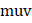# A ball is dropped from a height of 45 m from the ground. The coefficient of restitution between the ball and the ground is 2/3. What is the distance travelled by the ball in 4th second of its motion. Assume negligible time is spent in rebounding. Leta) 5 m b) 20 m c) 15 m d) 10 m

## Question ID - 100238 :- A ball is dropped from a height of 45 m from the ground. The coefficient of restitution between the ball and the ground is 2/3. What is the distance travelled by the ball in 4th second of its motion. Assume negligible time is spent in rebounding. Leta) 5 m b) 20 m c) 15 m d) 10 m

3537

(c)

Time taken to fall through 45 m =m/sDistance travelled in the fourth second=distance travelled in 1 s after reboundingNext Question :
 A particle of massis moving horizontally with a constant velocitytowards a rigid wall that is moving in opposite direction with a constant speed  . Assuming elastic impact between the particle and wall, the work done by the wall in reflecting the particle is equal to a) (1/2)b) (1/2)c) (1/2)d) None of these